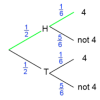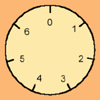# Introductions to ...### An Introduction to Magic Squares

##### Age 7 to 16### Divisibility Tests

##### Age 11 to 16

This article explains various divisibility rules and why they work. An article to read with pencil and paper handy.### An Introduction to Tree Diagrams

##### Age 11 to 16

This article explains how tree diagrams are constructed and helps you to understand how they can be used to calculate probabilities.### An Introduction to Modular Arithmetic

##### Age 14 to 18

An introduction to the notation and uses of modular arithmetic### An Introduction to Irrational Numbers

##### Age 14 to 18

Tim Rowland introduces irrational numbers### An Introduction to Proof by Contradiction

##### Age 14 to 18

An introduction to proof by contradiction, a powerful method of mathematical proof.